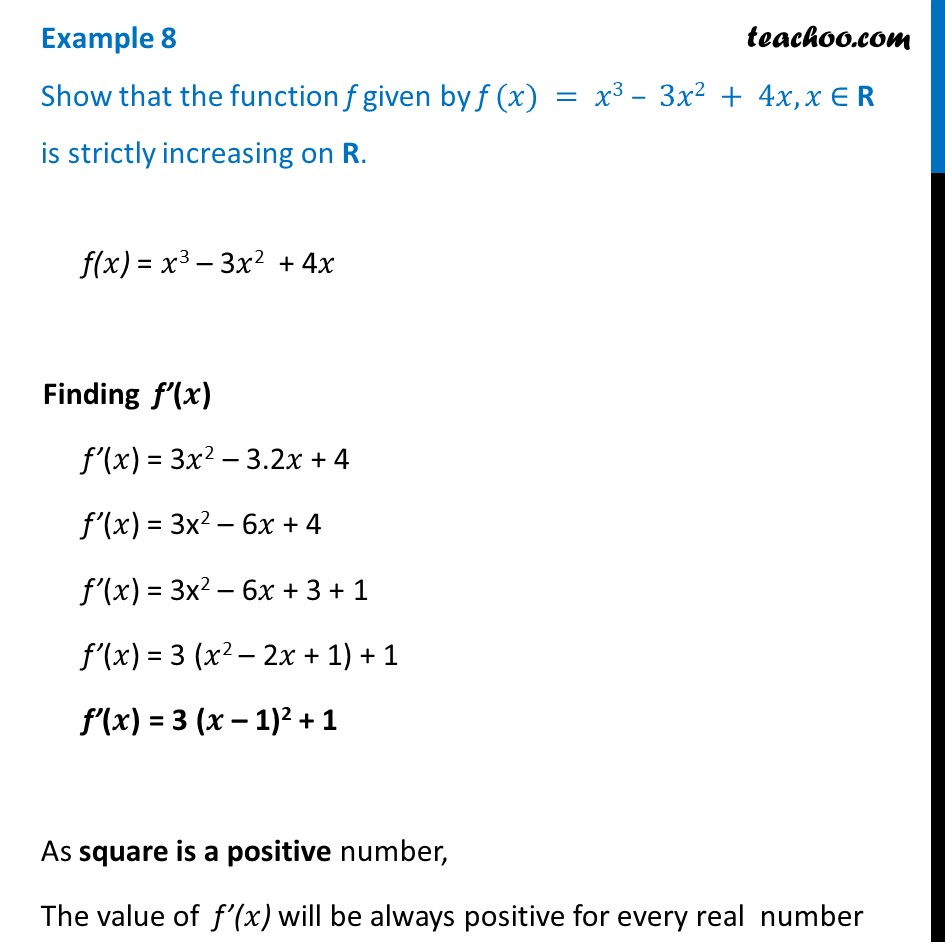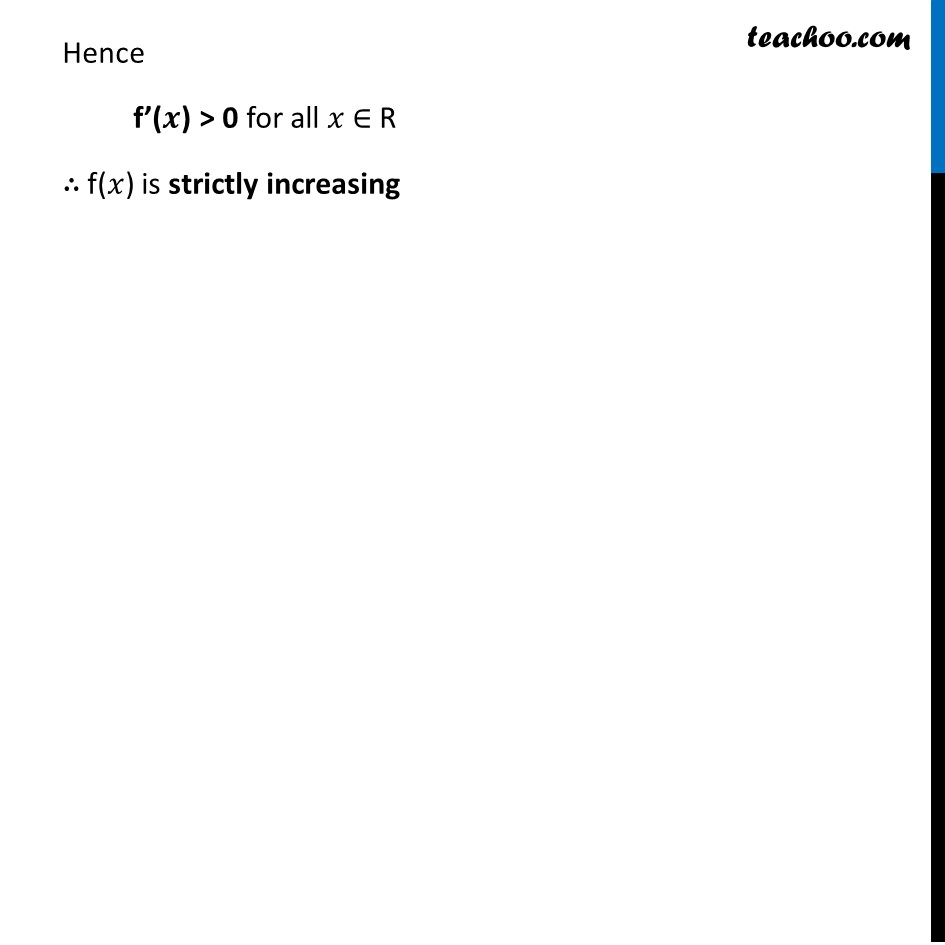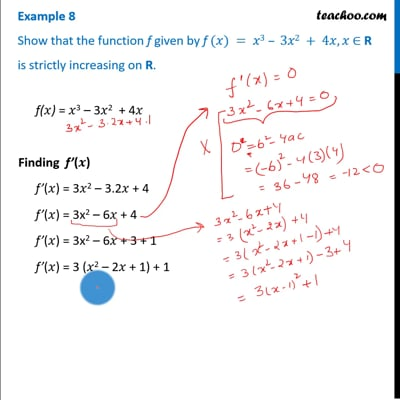Examples

Chapter 6 Class 12 Application of Derivatives
Serial order wiseThis video is only available for Teachoo black users

Solve all your doubts with Teachoo Black (new monthly pack available now!)

### Transcript

Example 8 Show that the function f given by f (𝑥) = 𝑥3 – 3𝑥2 + 4𝑥, 𝑥 ∈ R is strictly increasing on R. f(𝑥) = 𝑥3 – 3𝑥2 + 4𝑥 Finding f’(𝒙) f’(𝑥) = 3𝑥2 – 3.2𝑥 + 4 f’(𝑥) = 3x2 – 6𝑥 + 4 f’(𝑥) = 3x2 – 6𝑥 + 3 + 1 f’(𝑥) = 3 (𝑥2 – 2𝑥 + 1) + 1 f’(𝒙) = 3 (𝒙 – 1)2 + 1 As square is a positive number, The value of f’(𝑥) will be always positive for every real number Hence f’(𝒙) > 0 for all 𝑥 ∈ R ∴ f(𝑥) is strictly increasing